# 【20180806】【C/C++基础知识】指针与一维数组（访问、输出数组元素，指针与数组的首地址），指针与二维数组（行指针、列指针，访问、输出二维数组元素），指针数组处理多个字符串

16 篇文章 2 订阅

C编译程序用数组名存放数组在内存中的首地址。

### 指针与一维数组

• 指针移动方式访问数组元素：
int a, *p;     // a存放了数组的首地址
p=a;     // 数组首地址赋给p
p++;     // 向下移动4个字节

int *pa=a; 和 int *pa=&a;（// a的首地址）两者完全等价！

pa和a可以混合使用的图片说明，前面一列是地址（a和pa都表示地址），加*表示里面的元素。指针还可以移动，如图2。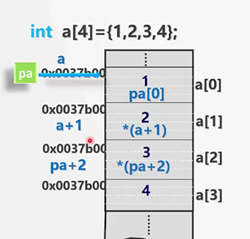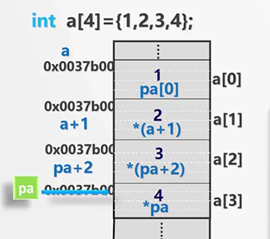• 数组元素的等价引用形式：

a[i]

pa[i]   // pa和a可以混合使用，pa和a都表示首地址

*(a+i)   // 表示地址的前面加一个*，就代表该地址里面的元素

*(pa+i)   // pa和a混合使用

• 对应的数组元素的地址表现形式：

&a[i]    // 加取地址符号就表示地址

&pa[i]

(a+i)    // *和&互为逆运算，因此前面没有了符号

(pa+i)

/* 用指针访问数组，计算数组的元素之和 */
#include<stdio.h>
#include<stdlib.h>
int main()
{
int iarray={0,2,4,6,8,10,12,14,16,18},*p=iarray;
int i,sum=0;
for(i=0;i<10;i++)
{
sum+=*p;
p++;        // sum+=*iarray; iarray++是错误的！数组名不能进行自增操作！
｝
printf("sum is %d\n",sum);
system("pause");
return 0;
}

• 用指针进行数组元素的输入输出（同样只能一次处理一个元素，用for循环）：

for(i=0;i<4;i++)

{

scanf_s(“%d”,&a[i]);   // 输入

printf(“%d”,a[i]);   // 输出

}

for(p=a;p<(a+4);p++)

{

scanf_s(“%d”,p);   // 用指针输入，p本身是个地址，不用再加取地址符号

printf(“%d”,*p);   // 用指针输出

}

• 访问数组元素的方法（五种，可混合使用）：

void main()    // 定义和初始化

{

int sum1,sum2,sum3,sum4,sum5;

int iarray[]={1,3,2,5,2,56,34,2,7,60};

int *iptr;

int size,n;

sum1=sum2=sum3=sum4=sum5=0;

size=sizeof(iarray)/sizeof(*iarray);

}

for(n=0;n<size;n++)

{

sum1+=iarray[n];

}

iptr=iarray;

for(n=0;n<size;n++)

{

sum2+=*iptr;

}

iptr=iarray;

for(n=0;n<size;n++)

{

sum3+=*(iptr+n);

}

iptr=iarray;

for(n=0;n<size;n++)

{

sum4+=iptr[n];

}

for(n=0;n<size;n++)

{

sum5+=*(iarray+n);

}

• 求数组的首地址（两种）：

• 通过数组首地址访问数组元素的方式：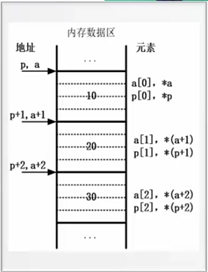### 指针与二维数组

C语言是按照行优先的原则存储数组的。

int a;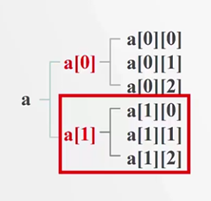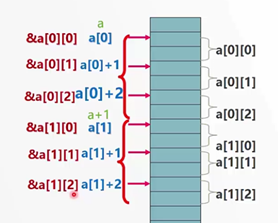• 二维数组与行：

(1) a代表二维数组的首地址，也是第0行的首地址；

(2) a+i代表第i行元素的首地址，即&a[i]；

(3) a+i或&a[i]表示行地址，每次加一表示会移动到下一行；

(4) *(a+i)即a[i]，不代表具体元素，依然是个地址（第i行的首地址）。

• 二维数组与列：

(1) 对于二维数组，第i个元素依然是一个一维数组，即*(a+i)即a[i]依然是一个地址；

(2) 对于每一个一维数组a[i]或*(a+i)，a[i]+j或*(a+i)+j表示该一维数组a[i]中第j个元素的地址，即&a[i][j]；

(3) *(a[i]+j)或*(*(a+i)+j)代表一维数组a[i]中第j个元素的值，即a[i][j]；

(4) a[i]或*(a+i)表示列地址，每次加一会移动一个元素。

• 二维数组的理解（行地址、列地址）：

(1) 有两行，a和a分别为两行的首地址；

(2) 每一行有四个元素；

(3) 对于每一行的地址是a+0，a+1，或&a，&a；

(4) a+0或&a对这一行是首地址，因为我们叫它“一维数组名a”，后面的中括号就是该一维数组名的下标。如下图：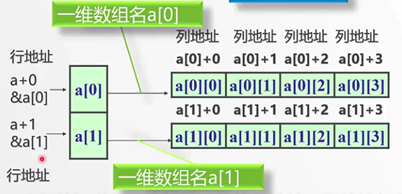• 行指针、列指针

1. 行指针：

int a;

int (*p);  // 定义一个行指针，指向一个数组，有四个元素。

p=a;   // 用行地址初始化

int (*p);

p=a;

for(i=0;i<m;i++)

for(j=0;j<n;j++)

printf(“%d”,p[i][]j);   // 下标的索引方式

2. 列指针：

int *p=*a;    // 用列地址初始化。

int *p=*a;   // 即p=*a; *a是第0行的首地址

for(i=0;i<m;i++)    // 行

for(j=0;j<n;j++)    // 列

printf(“%d”,*(p+i*n+j));    // 或下标索引：p[i*n+j]; 它是一个元素一个元素处理的。

(1) 二维数组首地址是行地址；

(2) 行指针指向二维数组名，行指针加减一，就移动一行；

(3) 行指针+i表示第i行的行地址，取*就转换为列地址，转为对一维数组的处理；

### 指针数组

int a;

int *p;

p=a;    // 错误！指针数组中没有这种初始化形式！只有行指针才能指向行地址！这里的p不是行指针！

int (*p);

p=a;    // 行指针指向二维数组行地址

int *p;    // 指针数组

(1) 由基类型相同的指针构成；

(2) 每个元素都是一个指针；

(3) 使用前必须初始化；

(4) 常用于对多个字符串进行处理。（一维字符数组可以处理一个字符串，那么二维字符数组可以存储和处理多个字符串）

char *proname[]={“FORTRAN”,”C”,”C++”};   // 定义一个指针数组并初始化

#define N 100   // 最多处理100个字符串

char *str[N];    // 指针数组

#define N 100   // 最多处理100个字符串

#define M 50   // 每个字符串长度不超过50

char str[N][M];    // 二维字符数组

(1) 一个是字符指针数组，每个元素都是用来存储字符串的地址。

(2) 字符串的长度可能不同，并且字符串之间可能没有关系，存储空间可能连续也可能不连续。

(3) 字符指针数组所需空间大小：100*4+90*2+10*50=1080（100个字符串的地址，每个地址占4个字节+字符串本身占据的空间）。二维字符数组所需空间：要求字符串长度最大为M，那么如果字符串长度超过M会发生越界，如果小于M，不会出错，但所需空间（1080）远小于我们产生的空间大小（5000），造成浪费。

(4) 字符指针数组元素指向的字符串可以是不规则的长度。字符二维数组的每个元素的长度必须相同，在定义时已确定。

(5) 因此可以看出，字符指针数组对空间的需求更少，更适合处理多个字符串。

09-15868
10-30967801-02983
07-2495
12-014323
05-082万+
09-16434
04-201万+
01-262438
01-151236
04-192015
05-3163
02-239716
12-19161
08-021万+
06-251万+
08-285065
08-292700点击重新获取扫码支付余额充值